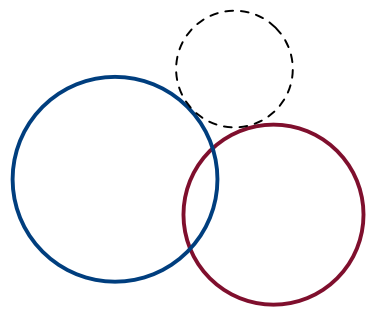# Locus-Pocus

Geometry Level 4Two non-congruent circles $\Gamma_1$ and $\Gamma_2$ intersect at two distinct points. The locus of the centers of all circles tangent to both $\Gamma_1$ and $\Gamma_2$ is...

×